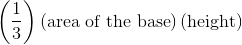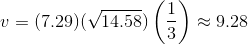PSAT Math : Pyramids

Example Questions

Example Question #1 : How To Find The Volume Of A Pyramid

The volume of a 6-foot-tall square pyramid is 8 cubic feet. How long are the sides of the base?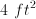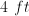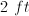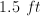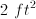Explanation:

Volume of a pyramid is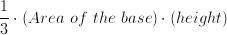Thus: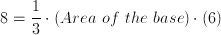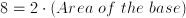Area of the base is.

Therefore, each side is.

Example Question #1 : How To Find The Volume Of A Pyramid

A right pyramid with a square base has a height that is twice the length of one edge of the base.  If the height of the pyramid is 6 meters, find the volume of the pyramid.

24

18

6

30

12

18

Explanation:

If the height, which is twice the length of the base edges, measures 6 meters, then each base edge must measure 3 meters.

Since the base is a square, the area of the base is 3 x 3 = 9.

Therefore the volume of the right pyramid is V = (1/3) x area of the base x height = 1/3(9)(6) = 18.

Example Question #1 : Pyramids

What is the approximate volume of a square pyramid with one edge of the base measuring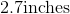and a height equal to the diagonal of its base?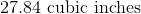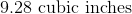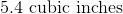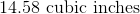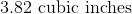If one edge of the base is 2.7 inches, and the height of the pyramid is equal to the diagonal of the base, we can find the height using the edge. The height is going to be equal to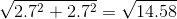. The area of the base is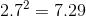. Volume of a pyramid is equal to